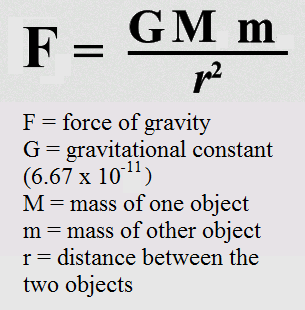1 like 0 dislike
270 views
What does the gravitational force equation explain?
| 270 views

4 like 0 dislike

This equation describes the force between any two objects in the universe:In the equation:

• F is the force of gravity (measured in Newtons, N)
• G is the gravitational constant of the universe and is always the same number
• M is the mass of one object (measured in kilograms, kg)
• m is the mass of the other object (measured in kilograms, kg)
• r is the distance those objects are apart (measured in meters, m)

So if you know how massive two objects are and how far they are apart, you can figure out the force between them.

by Wooden (426 points)
selected by
3 like 0 dislike
F = G . (M1.M2)/r2

Gravitational force equals the force of gravitational constant (G) times the Masses of the 2 objects divided by the distance between their centres squared.

SImilar to the equation for Coulomb's law, this explains the relationshioshp between distance, mass and force.
by Wooden (1.7k points)
2 like 0 dislike
Every object in the universe attracts every other 0bject.The gravitational force formula,also known as Newtons Law of Gravitational, defines the magnitude of the force between any two objects.The formula for the gravitational force includes the gravitational constant
by Wooden (864 points)
1 like 0 dislike
Force is directly proportional to the product of the two masses and inverseley proportional to the to the square of the distance between the point masses

F= (GMm) / r^2
by Wooden (1.1k points)
1 like 0 dislike

Newton's law of universal gravitation states that every point mass attracts every other point mass by a force acting along the line intersecting the two points. The force is proportional to the product of the two masses, and inversely proportional to the square of the distance between them.

The equation for universal gravitation thus takes the form:where F is the gravitational force acting between two objects, m1 and m2 are the masses of the objects, r is the distance between the centers of their masses, and G is the gravitational constant.

by Wooden (2k points)
0 like 0 dislike
Gravitational force is directly proportional to the mass of both interacting objects, more massive objects will attract each other with a greater Gravitational force, So as the mass of either objects increases, The force of gravitation attraction between them also increases.
by Wooden (1.5k points)
0 like 0 dislike

The gravitational Force equation describes the force between any two objects with mass in the universe:

F=  GMm/r^2

Where:

F is the force of gravity  measured in Newtons (N)

G  is the gravitational constant of the universe which is always constant

M is the mass of one object measured in kilograms (kg)

m is the mass of the other object measured in kilograms (kg)

r is the distance those objects are apart measured in meters (m)

by Wooden (381 points)

0 like 0 dislike
0 like 0 dislike